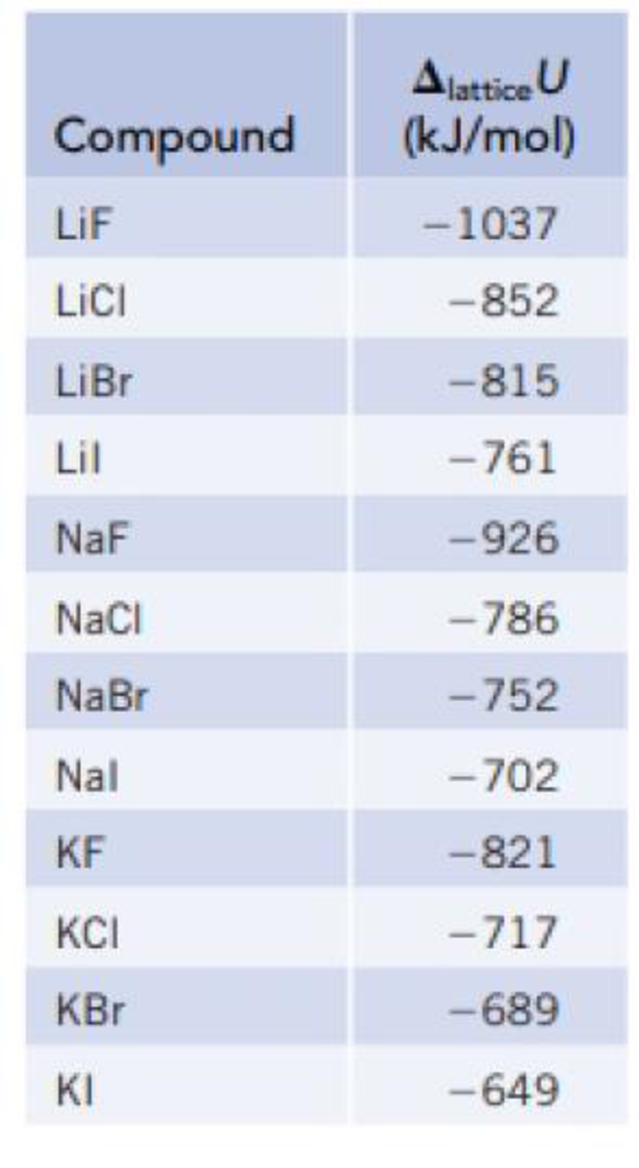Chapter 12, Problem 18PS

Chapter
Section
Textbook Problem

Calculate the lattice enthalpy for RbCl. In addition to data in Appendices F and L, you will need the following information;     Δ f H ° [Rb(g)] = 80 .9 kJ/mol Δ f H ° [RbCl(s)] =  − 435 .4 kJ/mol TABLE 12.1Lattice Energies of Some Ionic CompoundsInterpretation Introduction

Interpretation:

The lattice enthalpy of rubidium chloride has to be calculated.

Concept introduction:

The lattice enthalpy is calculated by using following method,

ΔHof(RbCl)=ΔH at(Rb)+ΔH at(Cl)+ΔHIE(Rb)+ΔHEA(Cl)+ΔHlattice(RbCl)

Explanation

The molar enthalpy formation is calculated by using Hess law, the enthalpy of the formation is given below

ΔHof(RbCl)=ΔH at(Rb)+ΔH at(Cl)+ΔHIE(Rb)+ΔHEA(Cl)+ΔHlattice(RbCl)ΔHof(RbCl)=-435.4kJ/molΔH at(Rb)=+80.9kJ/molΔH at(Cl)=+121kJ/molΔHIE(Rb)=+403kJ/molΔHEA

Still sussing out bartleby?

Check out a sample textbook solution.

See a sample solution

The Solution to Your Study Problems

Bartleby provides explanations to thousands of textbook problems written by our experts, many with advanced degrees!

Get Started